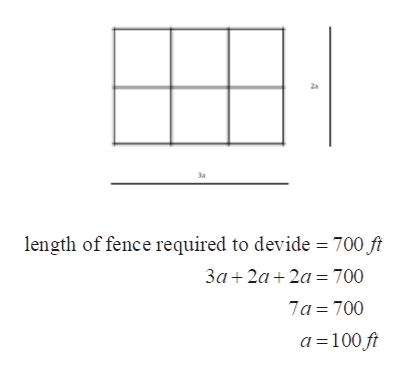# A farmer needs to take a rectangular plot of land and divide it into 6fields of equal area. He has 700 feet of fence available to divide up this plot of land. What will be the dimensions for one of the fields if he wants to maximize the total area of the 6 fields?

Question
68 views

A farmer needs to take a rectangular plot of land and divide it into 6
fields of equal area. He has 700 feet of fence available to divide up this plot of land. What will be the dimensions for one of the fields if he wants to maximize the total area of the 6 fields?

check_circle

Step 1

First of all, construct the diagram as per the question.

Let, l = length of the field.

b = breadth of the field.

The field is divided into six equal squares.

a = side of a square.

Step 2

Now, total length of the fence is 700 feet to divide the land into six equal square.

So, the total perimeter of the square will be considered excluding the exterior perimeter because fence is not used to surround the land.help_outlineImage Transcriptionclose3a length of fence required to devide 700 ft 3a 2a 2a 700 7a 700 a 100 ft fullscreen
Step 3

Now from diagram get the constrains.

And from the question get t...

### Want to see the full answer?

See Solution

#### Want to see this answer and more?

Solutions are written by subject experts who are available 24/7. Questions are typically answered within 1 hour.*

See Solution
*Response times may vary by subject and question.
Tagged in

### Calculus